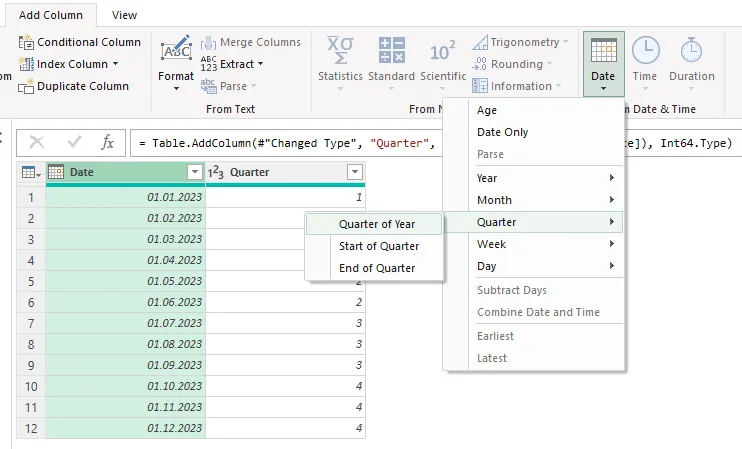# Calculate quarter in DAX from date using Power BI or Excel

Here is an approach that will help you calculate quarter in DAX from the date using various tools like Power BI or Excel data model. The previous post contains a method that is useful not only in Excel but also using Power BI.

## Quarter number in DAX

If you are using Power BI, you can get a quarter as a number from the date by using DAX function QUARTER.

`Quarter = QUARTER('Table'[Date])`

In the Excel data model, this function might not be available, and it is good to know how to get a quarter number no matter which tool you are using. Here is the universal approach.

```Quarter =
INT ( ( MONTH ( 'Table'[Date] ) + 2 ) / 3 )```

If you know the quarter number, you can do a couple of other calculations, as in this post below.

### Start of the quarter in DAX

```StartOfQuarter =
EOMONTH ( DATE ( YEAR ( 'Table'[Date] ), 'Table'[Quarter] * 3 - 3, 1 ), 0 ) + 1```

### End of the quarter in DAX

```EndOfQuarter =
EOMONTH ( DATE ( YEAR ( 'Table'[Date] ), 'Table'[Quarter] * 3, 1 ), 0 )```

### Year and quarter combination in Power BI

If you know how to combine text, you can create year and quarter combinations in many ways. Here is one of them.

```YearAndQuarter =
YEAR ( 'Table'[Date] ) & " Q" & 'Table'[Quarter]```

If you are doing data transformation in Power Query, you can select the date column, go to the Add Column tab and generate the necessary content.Thank you for reading this post, and please look at other DAX-related posts in this blog. There are a lot of similarities with Excel functions. Try to look at the differences even for the functions with the same name.

Posted

in